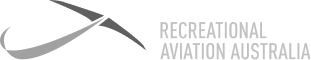# Definition: Centre of Gravity (CoG)

Is the position on the aircraft where it would balance on a point if it was possible to be suspended at that point. This is calculated by supporting the aircraft on a set of scales and noting the weights shown on each set.### Centre of Gravity (CoG)

Is the position on the aircraft where it would balance on a point if it was possible to be suspended at that point. This is calculated by supporting the aircraft on a set of scales and noting the weights shown on each set.

7 Day

FREE

Trial